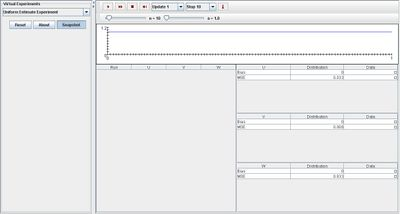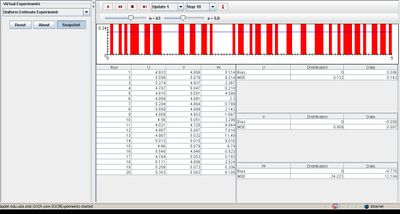# SOCR EduMaterials Activities UniformEstimateExperiment

(diff) ← Older revision | Latest revision (diff) | Newer revision → (diff)

## Description

The experiment is to generate a random sample X1, X2, ..., Xn of size n from the uniform distribution on (0, a). The distribution density is shown in blue in the graph, and on each update, the sample density is shown in red. On each update, the following statistics are recorded: U =2M where M = (X1 + X2 + ••• + Xn) / n (the sample mean)

V = (n + 1)X(n) / n where X(n) = max{X1, X2, ..., Xn} (the n'th order statistic)

W = (n + 1)X(1) where X(1) = min{X1, X2, ..., Xn} (the first order statistic)

## Goal

The purpose of the Uniform Estimate Experiment is to provide an applet that demonstrates types of functions that converges uniformly if the speed of convergence does not depend on the variable. After using this applet, one should be able to obtain the basic concepts of the function such as continuity, differentiability, and integral properties.

## Experiment

Go to the SOCR Experiment [SOCR Experiment] and select the Uniform Estimate Experiment from the drop-down list of experiments on the top left. The image below shows the initial view of this experiment:When pressing the play button, one trial will be executed and recorded in the distribution table below. The fast forward button symbolizes the nth number of trials to be executed each time. The stop button ceases any activity and is helpful when the experimenter chooses “continuous,” indicating an infinite number of events. The fourth button will reset the entire experiment, deleting all previous information and data collected. The “update” scroll indicates nth number of trials (1, 10, 100, or 1000) performed when selecting the fast forward button and the “stop” scroll indicates the maximum number of trials in the experiment. Modifying either parameter does not change the distribution graph of the events, but varying parameter a changes the values of the axes. For each trial, the sample density roughly illustrates the same values. The image below demonstrates this:## Applications

The Uniform Estimate Experiment is a simple application that can be used to help gather data information regarding mean and the first and nth order statistic. It may be used in many different types of events such as:

Suppose researchers want to demonstrate their findings of a uniform experiment involving men and women. They discover the results to be approximately uniform and use this applet to display the outcomes graphically.